Fall 2003

# Mathematics Math21b Fall 2003

## Linear Algebra and Differential Equations

Office: SciCtr 434
Email: knill@math.harvard.edu# The Tacoma Bridge

[An update from: January 1, 2016: the resonance picture of the Tacoma bridge had long been the standard explanation of the collapse. The article indicates that the resonance picture, which appears in many books and pages might not be correct. The Motherboard article (a previous Motherboard article had got it wrong first too) links to this page (which was written 12 years ago and is not modified besides where indicated. At the end is an modern physics Today article explaining more.]

DSolve[x''[t]+ k^2 x[t] -Cos[k t]==0, x[t], t]
1) Without force. The harmonic oscillator
 ``` x''(t) + k2 x(t) = 0 ```
has the solutions a cos(k t) + b sin(k t). The differential equation can be written as A x = 0, with the linear operator A=D2+k2. The solutions are in the kernel of A.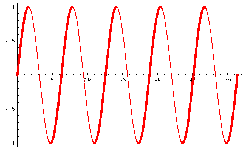2) Close to resonance. The problem with periodic forcing
 ``` x''(t) + k2 x(t) = cos(w t) ```
can be written as A f = b. If we find a special solution x, then the general solution will be of the form x + a cos(k t) + b sin(k t). A special solution is obtained with the "ansatz" (*) x(t) = c cos(w t) which gives c(-w2+k2) cos(w t) = cos(w t) or c=1/(k2-w2). The amplitude c of the special solution increases if the driving frequency w becomes close to the resonance frequency.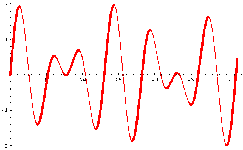3) In resonance. When w=k, the problem
 ``` x''(t) + k2 x(t) = cos(k t) ```
has the special solution x(t) = t sin(k t)/(2k).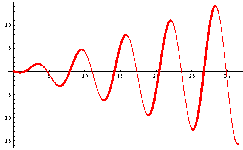(*) We learned how to solve this without funny cookbook methods. The ODE's P(D) x = g is solved by writing the "matrix" P(D)=(D-k1) ... (D-k1) as a product of matrices Ai=(D-ki) and because Ai f = g can be solved by an explicit formula, we can invert A by inverting one Ai after the other, each inversion introducing a new consant. This operator method always works and generalizes the case Dn f =g, where f is obtained by integrating g several times. The cookbook method has the advantage that it is faster but cookbooks usually don't tell why things are cooked that way.

The Tacoma Narrows Bridge in Washington state, was with 1.9 km length one of the largest suspended bridges built at the time. The bridge connecting the Tacoma Narrows channel collapsed in a dramatic way on Thursday November 7, 1940. Winds of 35-46 mi/hours =65-75 km/hr) produced an oscillation which eventually broke the construction.
Below you see some movies taken during that event. The bridge began first to vibrate the bridge torsinonally, giving it a twisting motion. Later the vibrations entered a natural resonance frequency of the bridge which started to increase their amplitude.
A car which had been left on the bridge could no more be saved. It contained an unfortunate dog who (so an other story) was so frightend that he would bite whoever would try to rescue him.

 The man on the picture is Leonard Coatsworth, a Tacoma newspaper editor who recalls: "Just as I drove past the towers, the bridge began to sway violently from side to side. Before I realized it, the tilt became so violent that I lost control of the car, jammed on the brakes and got out, only to be thrown onto my face against the curb. "Around me I could hear concrete cracking. I started to get my dog Tubby, but was thrown again before I could reach the car. The car itself began to slide from side to side of the roadway. "On hands and knees most of the time, I crawled 500 yards or more to the towers. My breath was coming in gasps. My knees were raw and bleeding, my hands bruised and swollen from gripping the concrete curb. Toward the last, I risked rising to my feet and running a few yards at a time. Safely back at the toll plaza, I saw the bridge in its final collapse and saw my car plunge into the Narrows."

The bridge had been called "Galloping Gertie" and attracted tourists who wanted to feel the sensation of crossing the rolling center span. Although there had been concerns about the bridge's stability, officials had been so confident that they considered canceling the insurance policies. A new and safer bridge was built in 1950 and is still in use today. It is said that the new nickname is "Sturdy Gertie".
The story of the Tacoma bridge is widelely in engeneering, physics and calculus classes to motivate the study of differential equations.

 Feedback of a reader on September 26, 2007: ```It is far more accurate to express the ODE as m x"(t) + c x' + k x = F[x, U] , where c is the inherent structural damping, F[x, U] is a functional of x depending on a parameter U, the (steady) wind speed. As a linear approximation, [*] F[x, U] = f(U) + k*(U) x + c*(U) x' , where f, k* and c* depend on the shape of the bridge (the shallow H cross sectional geometry of the Tacoma Narrows bridge was a spectacularly poor choice) as well as the wind speed, resulting in m x"(t) + (c - c*(U)) x' + (k - k*(U)) x = f(U) . "Divergence" occurs if k - k*(U) = 0 for some U, since then the equilibrium solution x_eq = f(U) / (k - k*(U)) is unbounded, and nonlinear terms must be included. "Flutter" or "galloping" occurs when the effective damping c - c*(U) becomes negative for some U, that is, it is a Hopf bifurcation and not a simple resonance phenomenon. The applicability of this to the Tacoma Narrows bridge collapse is important because the resonance explanation implies that the wind is blowing back and forth over the bridge at one of its natural frequencies (the second torsional mode is this case), whereas the Hopf bifurcation explanation only requires a steady wind of sufficient speed. Eric [*] Robert D. Blevins, "Flow-induced vibration," Krieger, 1993 or E. H. Dowell, "A Modern Course in Aeroelasticity," Kluwer, 2005. Eric Petersen ```

 An update from: January 1, 2016: the story is more interesting: the article links to this page as an example of how not to explain this bridge collapse. The claim is that there was no resonance behavior in the collapse on 9 Nov 1940. Thanks to John Palkovic who pointed out to us the following Physics today article, which gives an other explanation and the source for the resonance story.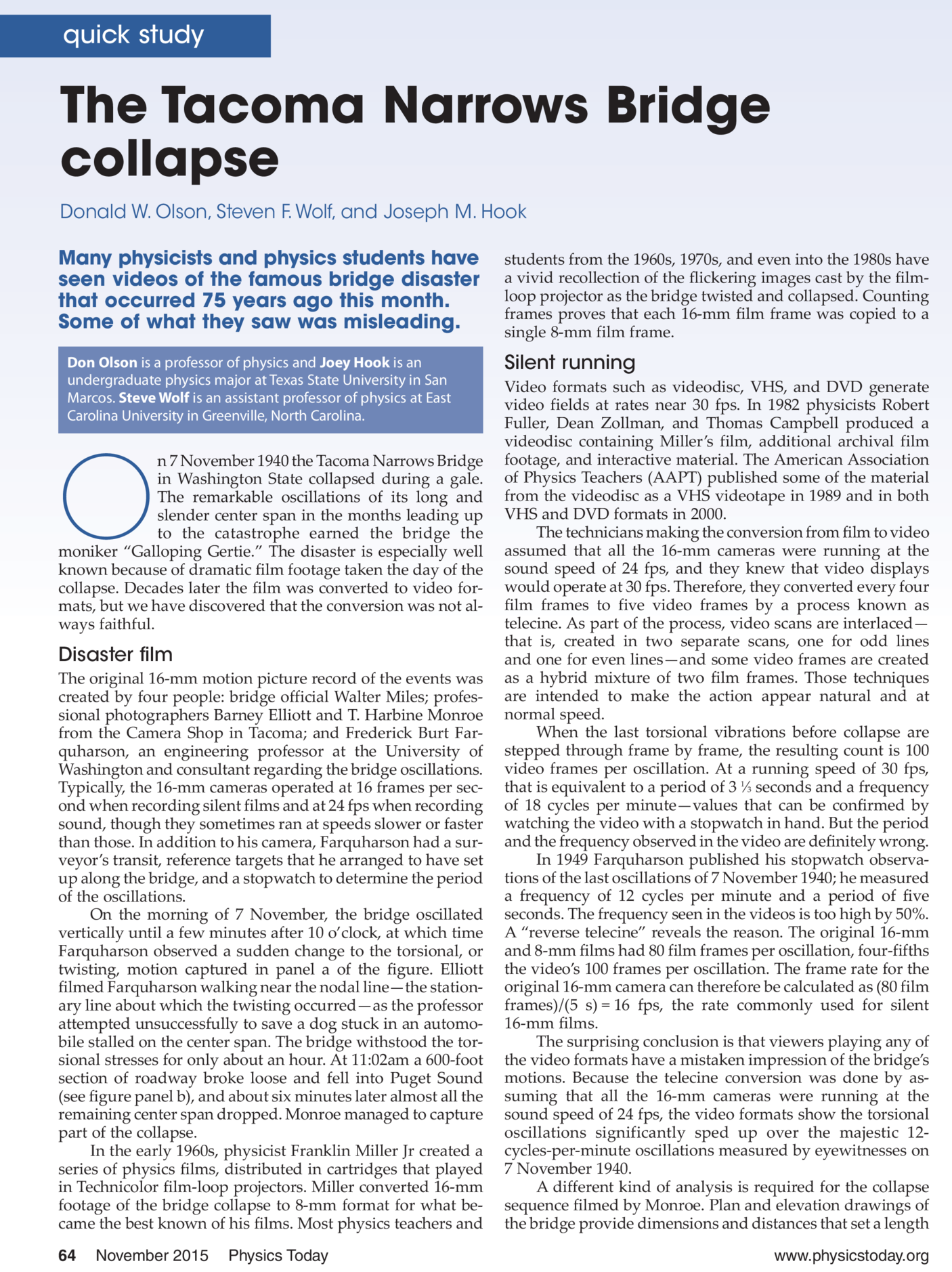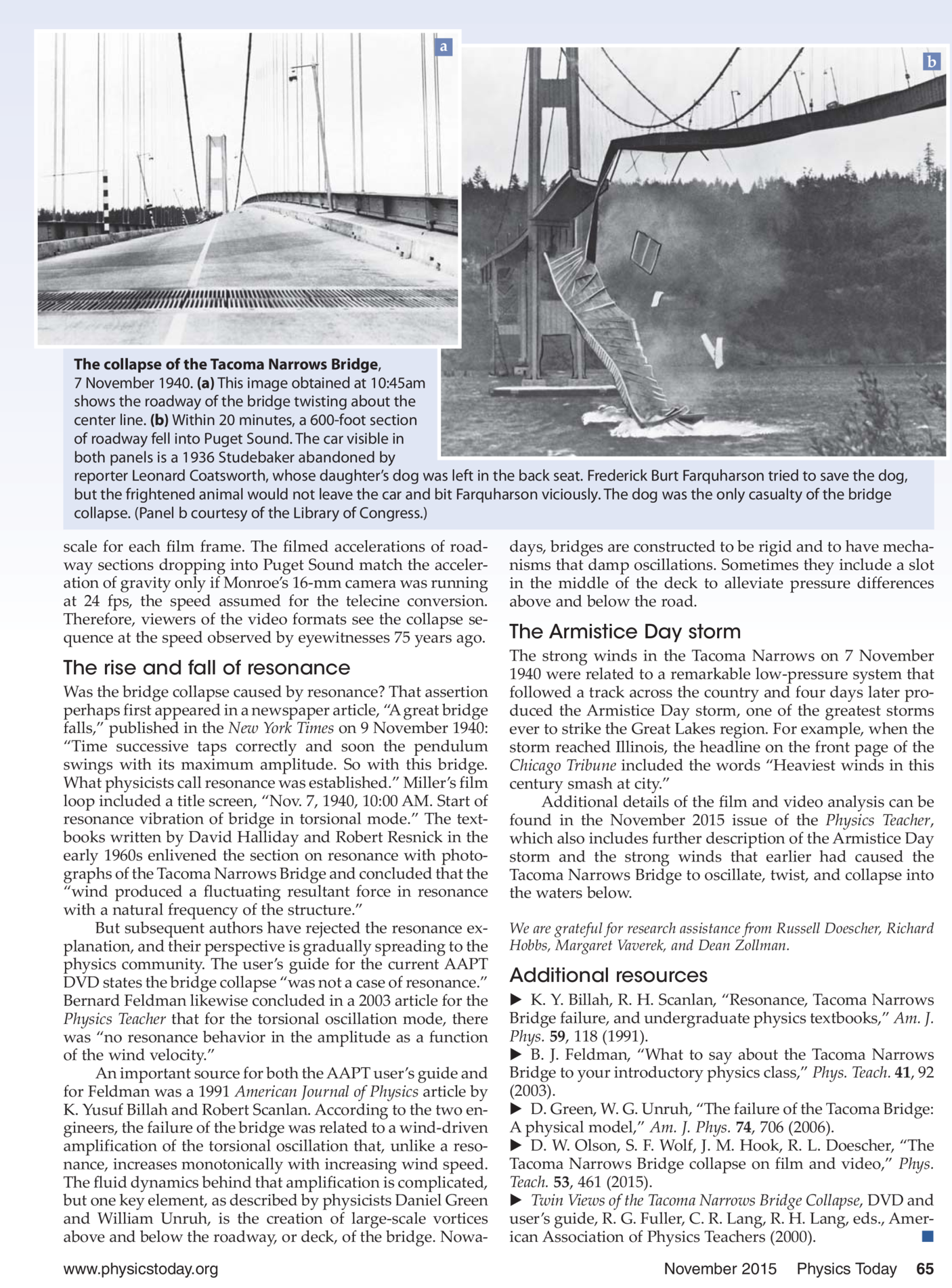Back to the main page